Zariski category

An abstract model of the category of commutative algebras (cf. Commutative algebra) in which basic commutative algebra and algebraic geometry can be performed. Zariski categories are axiomatically defined as categoriessatisfying the following six axioms:

1)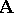is co-complete, i.e., has all small colimits;

2)has a strong generating set of objects whose objects are finitely presentable and flatly co-disjunctable;

3) regular epimorphisms inare universal, i.e., stable under pull-backs along any morphism (cf. Morphism);

4) the terminal object ofis finitely presentable and has no proper subobject;

5) the product of two objects inis co-universal, i.e., stable under pushouts along any morphism;

6) for any finite sequence of co-disjunctable congruences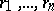on any object, with respective co-disjunctors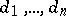, one has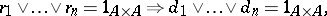where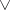denotes union of congruences onon the left-hand side and co-union of quotient objects ofon the right-hand side.

These axioms rely on the notions of limits, colimits, finitely presentable objects, and co-disjunctors [a1]. This latter notion describes in a universal way the calculus of fractions, and is defined as follows. Let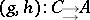be an arbitrary pair of parallel morphisms in. It is said to be co-disjointed if its co-equalizer is a strict terminal object. A morphismco-disjoints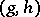if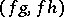is co-disjointed. A co-disjunctor ofis a morphism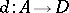that co-disjointsand through which any morphismthat co-disjointsfactors uniquely. The pairis co-disjunctable if it has a co-disjunctor. The objectis co-disjunctable if the pair of inductions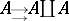ofinto its coproduct by itself is co-disjunctable. It is flatly co-disjunctable if, moreover, the co-disjunctorofis a flat morphism, i.e., the pushout functor alongpreserves monomorphisms.

The whole of basic commutative algebra and algebraic geometry can be formally developed in any Zariski category as if its objects were commutative rings. Any result proven in an arbitrary Zariski category has various interpretations in various concrete categories of commutative algebras of different kinds, possible equipped with some extra structure such as an order, lattice, gradation, filtration, differentiation, etc.

How to Cite This Entry:
Zariski category. Encyclopedia of Mathematics. URL: http://encyclopediaofmath.org/index.php?title=Zariski_category&oldid=24015
This article was adapted from an original article by Y. Diers (originator), which appeared in Encyclopedia of Mathematics - ISBN 1402006098. See original article